CGAL 5.5 - 2D Periodic Triangulations
CGAL::Periodic_2_triangulation_2< Traits, Tds > Class Template Reference

#include <CGAL/Periodic_2_triangulation_2.h>

## Definition

The class Periodic_2_triangulation_2 represents a 2-dimensional triangulation of a point set in $$\mathbb T_c^2$$.

Template Parameters
 Traits is the geometric traits, it has to be instantiated by a model of the concept Periodic_2TriangulationTraits_2. Tds is the triangulation data data structure and must be a model of TriangulationDataStructure_2 whose vertex and face are models of Periodic_2TriangulationVertexBase_2 and Periodic_2TriangulationFaceBase_2. It defaults to: CGAL::Periodic_2_triangulation_vertex_base_2,

Traversal of the Triangulation

The periodic triangulation class provides several iterators and circulators that allow one to traverse it.

I/O

The I/O operators are defined for iostream. The format for the iostream is an internal format.

The information in the iostream is:

• the original domain
• the number of sheets of the covering space as in number_of_sheets()
• the number of vertices
• the non-combinatorial information of vertices (point resp. point-offset pairs, etc.)
• the number of faces
• the indices of the vertices of each face
• the indices of the neighbors of each face, where the index corresponds to the preceding list of faces
• the offsets corresponding to the vertices of the faces
• the non-combinatorial information of each face

Implementation

Locate is implemented by a randomized walk from a vertex of the face given as optional parameter (or from an arbitrary vertex of if no optional parameter is given).

Insertion of a point is done by locating a face that contains the point, and then splitting this face. Apart from the location, insertion takes a time $$O(1)$$.

Removal of a vertex is more difficult than in the Euclidean space, since the star of a vertex may not be disjoint from the star of a virtual copy of that vertex. Therefore generic removal of vertices is not implemented. Several more constrained cases are implemented: the removal of the last vertex in the triangulation and the removal of a vertex of degree 3.

The face, edge, and vertex iterators on features are derived from their counterparts visiting all (non-virtual and virtual) features which are themselves derived from the corresponding iterators of the triangulation data structure.

CGAL::Periodic_2_triangulation_2<Traits,Tds>
CGAL::Periodic_2_triangulation_hierarchy_2<Tr>
CGAL::Triangulation_2<Traits, Tds>

## Public Types

enum  Iterator_type { STORED = 0, UNIQUE, STORED_COVER_DOMAIN, UNIQUE_COVER_DOMAIN }
The enum Iterator_type is defined by Periodic_2_triangulation_2 to specify the behavior of geometric iterators. More...

enum  Locate_type { VERTEX = 0, EDGE, FACE, EMPTY }
The enum @ is defined by Periodic_2_triangulation_2 to specify which case occurs when locating a point in the triangulation. More...

## Related Functions

(Note that these are not member functions.)

ostream & operator<< (ostream &os, const Periodic_2_triangulation_2< Traits, Tds > &t)
Writes the triangulation t into the stream os. More...

istream & operator>> (istream &is, Triangulation_2< Traits, Tds > &t)
Reads a triangulation from stream is and assigns it to t. More...

## Types

typedef Traits Geom_traits
the traits class.

typedef Tds Triangulation_data_structure
the triangulation data structure type.

typedef Geom_traits::Periodic_2_offset_2 Offset
the offset type.

typedef Geom_traits::Iso_rectangle_2 Iso_rectangle
the iso rectangle type.

typedef array< int, 2 > Covering_sheets
integer tuple to store the number of sheets in each direction of space.

typedef Geom_traits::Point_2 Point
the point type.

typedef Geom_traits::Segment_2 Segment
the segment type.

typedef Geom_traits::Triangle_2 Triangle
the triangle type.

typedef std::pair< Point, OffsetPeriodic_point
represents a point-offset pair. More...

typedef array< Periodic_point, 2 > Periodic_segment
a pair of periodic points representing a segment in the periodic domain.

typedef array< Periodic_point, 3 > Periodic_triangle
a triple of periodic points representing a triangle in the periodic domain.

typedef Tds::Vertex Vertex
the vertex type.

typedef Tds::Face Face
the face type.

typedef Tds::Edge Edge
the edge type.

typedef Tds::size_type size_type
size type (an unsigned integral type).

typedef Tds::difference_type difference_type
difference type (a signed integral type).

## Handles, Iterators and Circulators

The vertices and faces of the triangulations are accessed through handles, iterators and circulators.

The handles are CGAL models of the concept Handle which basically offers the two dereference operators and ->. The iterators and circulators are all bidirectional and non-mutable. The circulators and iterators are convertible to handles with the same value type, so that whenever a handle appear in the parameter list of a function, an appropriate iterator or circulator can be passed as well.

The edges of the triangulation can also be visited through iterators and circulators, the edge circulators and iterators are also bidirectional and non-mutable.

typedef Tds::Vertex_handle Vertex_handle
handle to a vertex.

typedef Tds::Face_handle Face_handle
handle to a face.

typedef Tds::Face_iterator Face_iterator
iterator over all faces.

typedef Tds::Edge_iterator Edge_iterator
iterator over all edges.

typedef Tds::Vertex_iterator Vertex_iterator
iterator over all vertices.

typedef unspecified_type Unique_vertex_iterator
iterator over the vertices whose corresponding points lie in the original domain, i.e. More...

typedef Face_iterator Finite_faces_iterator

typedef Edge_iterator Finite_edges_iterator

typedef Vertex_iterator Finite_vertices_iterator

typedef Face_iterator All_faces_iterator

typedef unspecified_type Face_circulator
circulator over all faces incident to a given vertex.

typedef unspecified_type Edge_circulator
circulator over all edges incident to a given vertex.

typedef unspecified_type Vertex_circulator
circulator over all vertices adjacent to a given vertex.

## Geometric iterators:

typedef unspecified_type Periodic_triangle_iterator
iterator over the triangles corresponding to faces of the triangulation.

typedef unspecified_type Periodic_segment_iterator
iterator over the segments corresponding to edges of the triangulation.

typedef unspecified_type Periodic_point_iterator
iterator over the points corresponding to vertices of the triangulation.

## Creation

Triangulation_2 (const Iso_rectangle &domain=Iso_rectangle(0, 0, 1, 1), const Geom_traits &traits=Geom_traits())
Introduces an empty triangulation t with domain as original domain. More...

Triangulation_2 (const Triangulation_2 &tr)
Copy constructor. More...

Triangulation_2 operator= (const Triangulation_2< Traits, Tds > &tr)
Assignment. More...

void swap (Triangulation_2 &tr)
The triangulations tr and this are swapped. More...

void clear ()
Deletes all faces and vertices resulting in an empty triangulation.

## Access Functions

The responsibility of keeping a valid triangulation belongs to the user when using advanced operations allowing a direct manipulation of the tds.

const Geom_traitsgeom_traits () const
Returns a const reference to the triangulation traits object.

const Triangulation_data_structure_2tds () const
Returns a const reference to the triangulation data structure.

Iso_rectangle domain () const
Returns the original domain.

Covering_sheets number_of_sheets () const
Returns the number of sheets of the covering space the triangulation is currently computed in.

int dimension () const
Returns the dimension of the convex hull. More...

size_type number_of_vertices () const
Returns the number of vertices. More...

size_type number_of_faces () const
Returns the number of faces. More...

size_type number_of_stored_vertices () const
Returns the number of vertices in the data structure. More...

size_type number_of_stored_faces () const
Returns the number of faces in the data structure. More...

## Non const access

This method is mainly a help for users implementing their own triangulation algorithms.

Triangulation_data_structure_2tds ()
This is an advanced function. More...

## Non-constant-time access functions

size_type number_of_edges () const
Returns the number of edges. More...

size_type number_of_stored_edges () const
Returns the number of edges in the data structure. More...

## Non-constant-time queries and conversions

It is not recommended to interfere with the built-in covering management.

Especially a premature conversion to the 1-sheeted covering space might lead to problems when modifying the triangulation later.

bool is_extensible_triangulation_in_1_sheet_h1 () const
This is an advanced function. More...

bool is_extensible_triangulation_in_1_sheet_h2 () const
This is an advanced function. More...

bool is_triangulation_in_1_sheet () const
This is an advanced function. More...

void convert_to_1_sheeted_covering ()
This is an advanced function. More...

void convert_to_9_sheeted_covering ()
This is an advanced function. More...

## Geometric access functions

Periodic_point periodic_point (const Vertex_handle v) const
Returns the periodic point given by vertex v. More...

Periodic_point periodic_point (const Face_handle f, int i) const
If this is represented in the 1-sheeted covering space, this function returns the periodic point given by the $$i$$-th vertex of face f, that is the point in the original domain and the offset of the vertex in f. More...

Periodic_segment periodic_segment (const Face_handle f, int i) const
Returns the periodic segment formed by the two point-offset pairs corresponding to the two vertices of edge (f,i). More...

Periodic_segment periodic_segment (const Edge &e) const
Same as the previous method for edge e.

Periodic_triangle periodic_triangle (const Face_handle f) const
Returns the periodic triangle formed by the three point-offset pairs corresponding to the three vertices of facet f.

Note that a traits class providing exact constructions should be used in order to guarantee the following operations to be exact (as opposed to computing the triangulation only, which requires only exact predicates).

Point point (const Periodic_point &pp) const
Converts the Periodic_point pp (point-offset pair) to the corresponding Point in $$\mathbb R^3$$.

Segment segment (const Periodic_segment &s) const
Converts the Periodic_segment s to a Segment.

Triangle triangle (const Periodic_triangle &t) const
Converts the Periodic_triangle this to a Triangle.

Segment segment (Face_handle f, int i) const
Equivalent to the call t.segment(t.periodic_segment(f,i));

Segment segment (const Edge &e) const
Equivalent to the call t.segment(t.periodic_segment(e));

Segment segment (const Edge_circulator &ec) const
Equivalent to the call t.segment(t.periodic_segment(ec->first, ec->second));

Segment segment (const Edge_iterator &ei) const
Equivalent to the call t.segment(t.periodic_segment(ei->first, ei->second));

Triangle triangle (Face_handle f) const
Equivalent to the call t.triangle(t.periodic_triangle(f));

## Predicates

The class Periodic_2_triangulation_2 provides methods to test the presence in the triangulation of a particular feature (edge or face).

bool is_edge (Vertex_handle va, Vertex_handle vb)
true if there is an edge having va and vb as vertices.

bool is_edge (Vertex_handle va, Vertex_handle vb, Face_handle &fr, int &i)
as above. More...

bool is_face (Vertex_handle v1, Vertex_handle v2, Vertex_handle v3)
true if there is a face having v1, v2 and v3 as vertices.

bool is_face (Vertex_handle v1, Vertex_handle v2, Vertex_handle v3, Face_handle &fr)
as above. More...

## Queries

The class Periodic_2_triangulation_2 provides methods to locate a given point with respect to a triangulation.

It also provides methods to locate a point with respect to a given face of the triangulation.

Face_handle locate (const Point &query, Face_handle f=Face_handle()) const
If the triangulation is not empty, a face that contains the query in its interior or on its boundary is returned. More...

Face_handle locate (const Point &query, Locate_type &lt, int &li, Face_handle h=Face_handle()) const
Same as above. More...

Oriented_side oriented_side (Face_handle f, const Point &p) const
Returns on which side of the oriented boundary of f the point p lies.

## Face, Edge and Vertex Iterators

The following iterators allow the user to visit faces, edges and vertices of the stored triangulation, i.e.

in case of computing in a multiply sheeted covering space all stored periodic copies of each item are returned. These iterators are non-mutable, bidirectional and their value types are respectively Face, Edge and Vertex. They are all invalidated by any change in the triangulation.

Vertex_iterator vertices_begin () const
Starts at an arbitrary vertex.

Vertex_iterator vertices_end () const
Past-the-end iterator.

Edge_iterator edges_begin () const
Starts at an arbitrary edge.

Edge_iterator edges_end () const
Past-the-end iterator.

Face_iterator faces_begin () const
Starts at an arbitrary face.

Face_iterator faces_end () const
Past-the-end iterator.

## Geometric iterators

The following iterators allow the user to obtain geometric primitives corresponding to faces, edges, and vertices of the triangulation.

These iterators are non-mutable, bidirectional and their value types are respectively Periodic_triangle, Periodic_segment and Periodic_point. They are all invalidated by any change in the triangulation. If the periodic triangulation is not computed in the 1-sheeted covering space, these iterators can be used to retain only the geometric primitives in the original domain. This can be controlled using the enum Iterator_type, see CGAL::Periodic_2_triangulation_2::Iterator_type.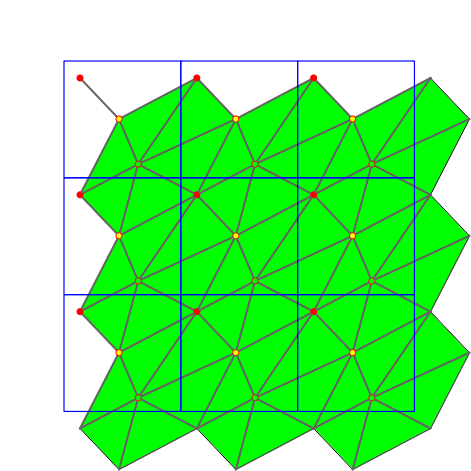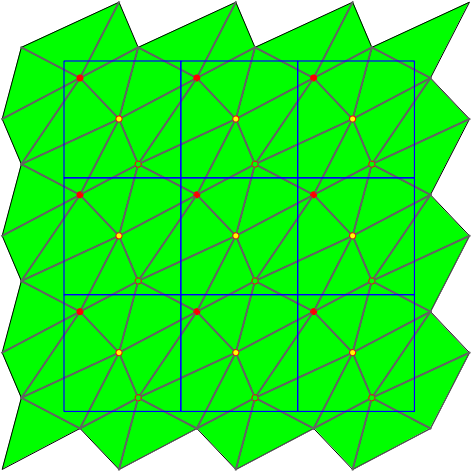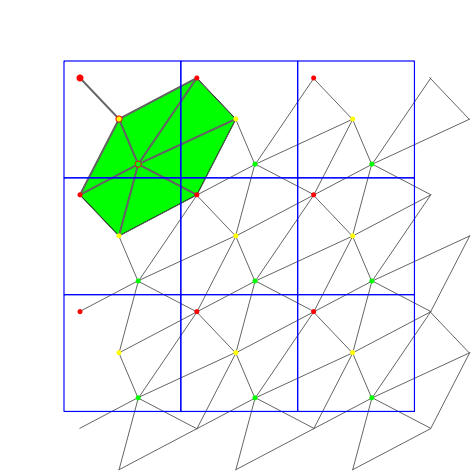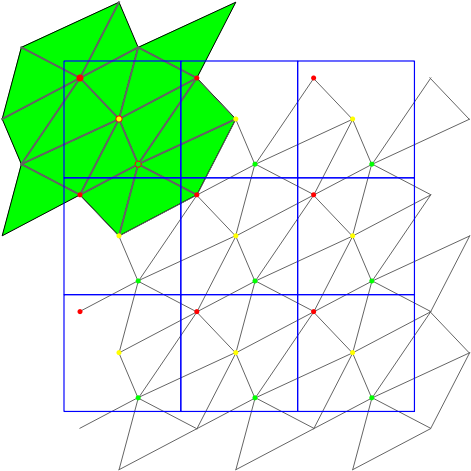The four different modes of the geometric iterators: STORED, STORED_COVER_DOMAIN, UNIQUE, UNIQUE_COVER_DOMAIN. Note that in case of computing in the 1-sheeted covering space, stored and unique give the same result.
Periodic_point_iterator periodic_points_begin (Iterator_type it=STORED) const
Iterates over the points of the triangulation. More...

Periodic_point_iterator periodic_points_end (Iterator_type it=STORED) const
Past-the-end iterator. More...

Periodic_segment_iterator periodic_segments_begin (Iterator_type it=STORED) const
Iterates over the segments of the triangulation. More...

Periodic_segment_iterator periodic_segments_end (Iterator_type it=STORED) const
Past-the-end iterator. More...

Periodic_triangle_iterator periodic_triangles_begin (Iterator_type it=STORED) const
Iterates over the triangles of the triangulation. More...

Periodic_triangle_iterator periodic_triangles_end (Iterator_type it=STORED) const
Past-the-end iterator. More...

## Face, Edge and Vertex Circulators

The triangulation also provides circulators that allows to visit respectively all faces or edges incident to a given vertex or all vertices adjacent to a given vertex.

These circulators are non-mutable and bidirectional. The operator++ moves the circulator counterclockwise around the vertex while the operator- moves clockwise. A face circulator is invalidated by any modification of the face pointed to. An edge or a vertex circulator are invalidated by any modification of one of the two faces incident to the edge pointed to.

Face_circulator incident_faces (Vertex_handle v) const
Starts at an arbitrary face incident to v.

Face_circulator incident_faces (Vertex_handle v, Face_handle f) const
Starts at face f. More...

Edge_circulator incident_edges (Vertex_handle v) const
Starts at an arbitrary edge incident to v.

Edge_circulator incident_edges (Vertex_handle v, Face_handle f) const
Starts at the first edge of f incident to v, in counterclockwise order around v. More...

Vertex_circulator adjacent_vertices (Vertex_handle v) const
Starts at an arbitrary vertex adjacent to v.

Vertex_circulator adjacent_vertices (Vertex_handle v, Face_handle f)
Starts at the first vertex of f adjacent to v in counterclockwise order around v. More...

## Traversal between adjacent faces

Vertex_handle mirror_vertex (Face_handle f, int i) const
returns the vertex of the $$i^{th}$$ neighbor of f that is opposite to f. More...

int mirror_index (Face_handle f, int i) const
returns the index of f in its $$i^{th}$$ neighbor. More...

Vertex_handle insert (const Point &p, Face_handle f=Face_handle())

Vertex_handle insert (const Point &p, Locate_type lt, Face_handle loc, int li)

Vertex_handle push_back (const Point &p)

template<class InputIterator >
int insert (InputIterator first, InputIterator last)

The following member functions offer more specialized versions of the insertion or removal operations to be used when one knows to be in the corresponding case.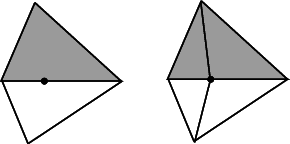Insertion of a point on an edge.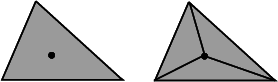Insertion in a face.

The following functions are mainly intended to be used in conjunction with the find_conflicts() member functions of Delaunay and constrained Delaunay triangulations to perform insertions.

Vertex_handle insert_first (const Point &p)
This is an advanced function. More...

Vertex_handle insert_in_face (const Point &p, Face_handle f)
This is an advanced function. More...

void remove_degree_3 (Vertex_handle v)
This is an advanced function. More...

void remove_first (Vertex_handle v)
This is an advanced function. More...

template<class EdgeIt >
Vertex_handle star_hole (Point p, EdgeIt edge_begin, EdgeIt edge_end)
This is an advanced function. More...

template<class EdgeIt , class FaceIt >
Vertex_handle star_hole (Point p, EdgeIt edge_begin, EdgeIt edge_end, FaceIt face_begin, FaceIt face_end)
This is an advanced function. More...

void set_domain (const Iso_rectangle dom)
This is an advanced function. More...

## Miscellaneous

int ccw (int i) const
Returns $$i+1$$ modulo 3. More...

int cw (int i) const
Returns $$i+2$$ modulo 3. More...

void flippable (Face_handle f, int i)

size_t degree (Vertex_handle v)
Returns the degree of the vertex v

## Checking

The responsibility of keeping a valid triangulation belongs to the users if advanced operations are used.

Obviously the advanced user, who implements higher levels operations may have to make a triangulation invalid at some times. The following method is provided to help the debugging.

bool is_valid (bool verbose=false, int level=0) const
This is an advanced function. More...

## Additional Inherited MembersPublic Member Functions inherited from CGAL::Triangulation_cw_ccw_2
Triangulation_cw_ccw_2 ()

int ccw (const int i) const

int cw (const int i) const

## ◆ Periodic_point

template<typename Traits , typename Tds >
 typedef std::pair< Point, Offset > CGAL::Periodic_2_triangulation_2< Traits, Tds >::Periodic_point

represents a point-offset pair.

The point in the pair lies in the original domain.

## ◆ Unique_vertex_iterator

template<typename Traits , typename Tds >
 typedef unspecified_type CGAL::Periodic_2_triangulation_2< Traits, Tds >::Unique_vertex_iterator

iterator over the vertices whose corresponding points lie in the original domain, i.e.

for each set of periodic copies the Unique_vertex_iterator iterates over exactly one representative.

## ◆ Iterator_type

template<typename Traits , typename Tds >

The enum Iterator_type is defined by Periodic_2_triangulation_2 to specify the behavior of geometric iterators.

Enumerator
STORED

Return all geometric primitives as they are stored internally in Triangulation_data_structure_2.

UNIQUE

Return only one representative of each geometric primitive even if the triangulation is computed in a multiply sheeted covering space.

Choose the representative whose maximum offset is minimal but non-negative in each direction of space.

STORED_COVER_DOMAIN

Same as STORED but return additionally all primitives whose intersection with the original domain of the current covering space is non-empty.

UNIQUE_COVER_DOMAIN

Same as UNIQUE but return additionally all primitives whose intersection with the original domain is non-empty.

## ◆ Locate_type

template<typename Traits , typename Tds >

The enum @ is defined by Periodic_2_triangulation_2 to specify which case occurs when locating a point in the triangulation.

If the triangulation does not contain any points EMPTY is returned.

Enumerator
VERTEX

when the located point coincides with a vertex of the triangulation

EDGE

when the point is in the relative interior of an edge

FACE

when the point is in the interior of a facet

EMPTY

when the triangulation is empty

## Member Function Documentation

template<typename Traits , typename Tds >
 Vertex_circulator CGAL::Periodic_2_triangulation_2< Traits, Tds >::adjacent_vertices ( Vertex_handle v, Face_handle f )

Starts at the first vertex of f adjacent to v in counterclockwise order around v.

Precondition
Face f is incident to vertex v.

## ◆ ccw()

template<typename Traits , typename Tds >
 int CGAL::Periodic_2_triangulation_2< Traits, Tds >::ccw ( int i ) const

Returns $$i+1$$ modulo 3.

Precondition
$$0 \leq i \leq 2$$.

## ◆ convert_to_1_sheeted_covering()

template<typename Traits , typename Tds >
 void CGAL::Periodic_2_triangulation_2< Traits, Tds >::convert_to_1_sheeted_covering ( )

This is an advanced function.

Converts the current triangulation into the same periodic triangulation in the 1-sheeted covering space.

Precondition
is_triangulation_in_1_sheet()

## ◆ convert_to_9_sheeted_covering()

template<typename Traits , typename Tds >
 void CGAL::Periodic_2_triangulation_2< Traits, Tds >::convert_to_9_sheeted_covering ( )

This is an advanced function.

Converts the current triangulation into the same periodic triangulation in the 9-sheeted covering space.

## ◆ cw()

template<typename Traits , typename Tds >
 int CGAL::Periodic_2_triangulation_2< Traits, Tds >::cw ( int i ) const

Returns $$i+2$$ modulo 3.

Precondition
$$0 \leq i \leq 2$$.

## ◆ dimension()

template<typename Traits , typename Tds >
 int CGAL::Periodic_2_triangulation_2< Traits, Tds >::dimension ( ) const

Returns the dimension of the convex hull.

The dimension is zero if the triangulation is empty and two otherwise.

## ◆ incident_edges()

template<typename Traits , typename Tds >
 Edge_circulator CGAL::Periodic_2_triangulation_2< Traits, Tds >::incident_edges ( Vertex_handle v, Face_handle f ) const

Starts at the first edge of f incident to v, in counterclockwise order around v.

Precondition
Face f is incident to vertex v.

## ◆ incident_faces()

template<typename Traits , typename Tds >
 Face_circulator CGAL::Periodic_2_triangulation_2< Traits, Tds >::incident_faces ( Vertex_handle v, Face_handle f ) const

Starts at face f.

Precondition
Face f is incident to vertex v.

## ◆ insert_first()

template<typename Traits , typename Tds >
 Vertex_handle CGAL::Periodic_2_triangulation_2< Traits, Tds >::insert_first ( const Point & p )

This is an advanced function.

Inserts the first vertex.

## ◆ insert_in_face()

template<typename Traits , typename Tds >
 Vertex_handle CGAL::Periodic_2_triangulation_2< Traits, Tds >::insert_in_face ( const Point & p, Face_handle f )

This is an advanced function.

Inserts vertex v in face f. Face f is modified, two new faces are created. If the triangulation contains periodic copies, a point is inserted in all periodic copies.

Precondition
The point in vertex v lies inside face f.

## ◆ is_edge()

template<typename Traits , typename Tds >
 bool CGAL::Periodic_2_triangulation_2< Traits, Tds >::is_edge ( Vertex_handle va, Vertex_handle vb, Face_handle & fr, int & i )

as above.

In addition, if true is returned, the edge with vertices va and vb is the edge e=(fr,i) where fr is a handle to the face incident to e and on the right side of e oriented from va to vb.

## ◆ is_extensible_triangulation_in_1_sheet_h1()

template<typename Traits , typename Tds >
 bool CGAL::Periodic_2_triangulation_2< Traits, Tds >::is_extensible_triangulation_in_1_sheet_h1 ( ) const

This is an advanced function.

The current triangulation remains a triangulation in the 1-sheeted covering space even after adding points if this method returns true. This test relies on a heuristic, i.e. if it answers false nothing is known. This test runs in constant-time when not computing in the 1-sheeted covering space. (This test uses the length of the longest edge in the triangulation as a criterion .)

## ◆ is_extensible_triangulation_in_1_sheet_h2()

template<typename Traits , typename Tds >
 bool CGAL::Periodic_2_triangulation_2< Traits, Tds >::is_extensible_triangulation_in_1_sheet_h2 ( ) const

This is an advanced function.

The same as is_extensible_triangulation_in_1_sheet_h1() but with a more precise heuristic, i.e. it might answer true in cases in which is_extensible_triangulation_in_1_sheet_h1() would not. However, it is much less time efficient when not computing in the 1-sheeted covering space. (This test uses the diameter of the largest empty circle in the input point set as a criterion .)

## ◆ is_face()

template<typename Traits , typename Tds >
 bool CGAL::Periodic_2_triangulation_2< Traits, Tds >::is_face ( Vertex_handle v1, Vertex_handle v2, Vertex_handle v3, Face_handle & fr )

as above.

In addition, if true is returned, fr is a handle to the face with v1, v2 and v3 as vertices.

## ◆ is_triangulation_in_1_sheet()

template<typename Traits , typename Tds >
 bool CGAL::Periodic_2_triangulation_2< Traits, Tds >::is_triangulation_in_1_sheet ( ) const

This is an advanced function.

Returns true if the current triangulation would still be a triangulation in the 1-sheeted covering space, returns false otherwise.

## ◆ is_valid()

template<typename Traits , typename Tds >
 bool CGAL::Periodic_2_triangulation_2< Traits, Tds >::is_valid ( bool verbose = false, int level = 0 ) const

This is an advanced function.

Checks the combinatorial validity of the triangulation and also the validity of its geometric embedding. This method is mainly a debugging help for the users of advanced features.

## ◆ locate() [1/2]

template<typename Traits , typename Tds >
 Face_handle CGAL::Periodic_2_triangulation_2< Traits, Tds >::locate ( const Point & query, Face_handle f = Face_handle() ) const

If the triangulation is not empty, a face that contains the query in its interior or on its boundary is returned.

If the triangulation is empty, the default constructed Face_handle is returned.

## ◆ locate() [2/2]

template<typename Traits , typename Tds >
 Face_handle CGAL::Periodic_2_triangulation_2< Traits, Tds >::locate ( const Point & query, Locate_type & lt, int & li, Face_handle h = Face_handle() ) const

Same as above.

Additionally, the parameters lt and li describe where the query point is located. The variable lt is set to the locate type of the query. If lt==VERTEX the variable li is set to the index of the vertex, and if lt==EDGE li is set to the index of the vertex opposite to the edge. Be careful that li has no meaning when the query type is FACE or when the triangulation is $$0$$-dimensional.

## ◆ mirror_index()

template<typename Traits , typename Tds >
 int CGAL::Periodic_2_triangulation_2< Traits, Tds >::mirror_index ( Face_handle f, int i ) const

returns the index of f in its $$i^{th}$$ neighbor.

Precondition
$$0 \leq i \leq 2$$.

## ◆ mirror_vertex()

template<typename Traits , typename Tds >
 Vertex_handle CGAL::Periodic_2_triangulation_2< Traits, Tds >::mirror_vertex ( Face_handle f, int i ) const

returns the vertex of the $$i^{th}$$ neighbor of f that is opposite to f.

Precondition
$$0 \leq i \leq 2$$.

## ◆ number_of_edges()

template<typename Traits , typename Tds >
 size_type CGAL::Periodic_2_triangulation_2< Traits, Tds >::number_of_edges ( ) const

Returns the number of edges.

Counts all edges that are representatives of the same segment in $$\mathbb T_c^2$$ as one edge.

## ◆ number_of_faces()

template<typename Traits , typename Tds >
 size_type CGAL::Periodic_2_triangulation_2< Traits, Tds >::number_of_faces ( ) const

Returns the number of faces.

Counts all faces that are representatives of the same triangle in $$\mathbb T_c^2$$ as one face.

## ◆ number_of_stored_edges()

template<typename Traits , typename Tds >
 size_type CGAL::Periodic_2_triangulation_2< Traits, Tds >::number_of_stored_edges ( ) const

Returns the number of edges in the data structure.

This is the same as the number of sheets times number_of_edges().

## ◆ number_of_stored_faces()

template<typename Traits , typename Tds >
 size_type CGAL::Periodic_2_triangulation_2< Traits, Tds >::number_of_stored_faces ( ) const

Returns the number of faces in the data structure.

This is the same as the number of sheets times number_of_faces().

## ◆ number_of_stored_vertices()

template<typename Traits , typename Tds >
 size_type CGAL::Periodic_2_triangulation_2< Traits, Tds >::number_of_stored_vertices ( ) const

Returns the number of vertices in the data structure.

This is the same as the number of sheets times number_of_vertices().

## ◆ number_of_vertices()

template<typename Traits , typename Tds >
 size_type CGAL::Periodic_2_triangulation_2< Traits, Tds >::number_of_vertices ( ) const

Returns the number of vertices.

Counts all vertices that are representatives of the same point in $$\mathbb T_c^2$$ as one vertex.

## ◆ operator=()

template<typename Traits , typename Tds >
 Triangulation_2 CGAL::Periodic_2_triangulation_2< Traits, Tds >::operator= ( const Triangulation_2< Traits, Tds > & tr )

Assignment.

All the vertices and faces are duplicated. After the assignment, this and tr refer to different triangulations: if tr is modified, this is not.

## ◆ periodic_point() [1/2]

template<typename Traits , typename Tds >
 Periodic_point CGAL::Periodic_2_triangulation_2< Traits, Tds >::periodic_point ( const Vertex_handle v ) const

Returns the periodic point given by vertex v.

If this is represented in the 1-sheeted covering space, the offset is always zero. Otherwise v can correspond to a periodic copy outside the domain of an input point.

## ◆ periodic_point() [2/2]

template<typename Traits , typename Tds >
 Periodic_point CGAL::Periodic_2_triangulation_2< Traits, Tds >::periodic_point ( const Face_handle f, int i ) const

If this is represented in the 1-sheeted covering space, this function returns the periodic point given by the $$i$$-th vertex of face f, that is the point in the original domain and the offset of the vertex in f.

If this is represented in the 9-sheeted covering space, this offset is possibly added to another offset determining the periodic copy.

Precondition
$$i \in\{0,1,2\}$$

## ◆ periodic_points_begin()

template<typename Traits , typename Tds >
 Periodic_point_iterator CGAL::Periodic_2_triangulation_2< Traits, Tds >::periodic_points_begin ( Iterator_type it = STORED ) const

Iterates over the points of the triangulation.

Its behavior is defined by the Iterator_type it as described on CGAL::Periodic_2_triangulation_2::Iterator_type.

## ◆ periodic_points_end()

template<typename Traits , typename Tds >
 Periodic_point_iterator CGAL::Periodic_2_triangulation_2< Traits, Tds >::periodic_points_end ( Iterator_type it = STORED ) const

Past-the-end iterator.

Note that to match another Periodic_point_iterator both must have the same Iterator_type it.

## ◆ periodic_segment()

template<typename Traits , typename Tds >
 Periodic_segment CGAL::Periodic_2_triangulation_2< Traits, Tds >::periodic_segment ( const Face_handle f, int i ) const

Returns the periodic segment formed by the two point-offset pairs corresponding to the two vertices of edge (f,i).

Precondition
$$i \in\{0,1,2\}$$

## ◆ periodic_segments_begin()

template<typename Traits , typename Tds >
 Periodic_segment_iterator CGAL::Periodic_2_triangulation_2< Traits, Tds >::periodic_segments_begin ( Iterator_type it = STORED ) const

Iterates over the segments of the triangulation.

Its behavior is defined by the Iterator_type it as described on CGAL::Periodic_2_triangulation_2::Iterator_type.

## ◆ periodic_segments_end()

template<typename Traits , typename Tds >
 Periodic_segment_iterator CGAL::Periodic_2_triangulation_2< Traits, Tds >::periodic_segments_end ( Iterator_type it = STORED ) const

Past-the-end iterator.

Note that to match another Periodic_segment_iterator both must have the same Iterator_type it.

## ◆ periodic_triangles_begin()

template<typename Traits , typename Tds >
 Periodic_triangle_iterator CGAL::Periodic_2_triangulation_2< Traits, Tds >::periodic_triangles_begin ( Iterator_type it = STORED ) const

Iterates over the triangles of the triangulation.

Its behavior is defined by the Iterator_type it as described on CGAL::Periodic_2_triangulation_2::Iterator_type.

## ◆ periodic_triangles_end()

template<typename Traits , typename Tds >
 Periodic_triangle_iterator CGAL::Periodic_2_triangulation_2< Traits, Tds >::periodic_triangles_end ( Iterator_type it = STORED ) const

Past-the-end iterator.

Note that to match another Periodic_triangle_iterator both must have the same Iterator_type it.

## ◆ remove_degree_3()

template<typename Traits , typename Tds >
 void CGAL::Periodic_2_triangulation_2< Traits, Tds >::remove_degree_3 ( Vertex_handle v )

This is an advanced function.

Removes a vertex of degree three. Two of the incident faces are destroyed, the third one is modified.

Precondition
Vertex v is a vertex with degree three.

## ◆ remove_first()

template<typename Traits , typename Tds >
 void CGAL::Periodic_2_triangulation_2< Traits, Tds >::remove_first ( Vertex_handle v )

This is an advanced function.

Removes the unique vertex in the triangulation.

## ◆ set_domain()

template<typename Traits , typename Tds >
 void CGAL::Periodic_2_triangulation_2< Traits, Tds >::set_domain ( const Iso_rectangle dom )

This is an advanced function.

Changes the domain. Note that this function calls clear(), i.e., it erases the existing triangulation.

## ◆ star_hole() [1/2]

template<typename Traits , typename Tds >
template<class EdgeIt >
 Vertex_handle CGAL::Periodic_2_triangulation_2< Traits, Tds >::star_hole ( Point p, EdgeIt edge_begin, EdgeIt edge_end )

This is an advanced function.

creates a new vertex v and use it to star the hole whose boundary is described by the sequence of edges [edge_begin, edge_end]. Returns a handle to the new vertex.

Precondition
The triangulation is a triangulation of 1 sheet

## ◆ star_hole() [2/2]

template<typename Traits , typename Tds >
template<class EdgeIt , class FaceIt >
 Vertex_handle CGAL::Periodic_2_triangulation_2< Traits, Tds >::star_hole ( Point p, EdgeIt edge_begin, EdgeIt edge_end, FaceIt face_begin, FaceIt face_end )

This is an advanced function.

same as above, except that the algorithm first recycles faces in the sequence [face_begin, face_end] and create new ones only when the sequence is exhausted.

Precondition
The triangulation is a triangulation of 1 sheet

## ◆ swap()

template<typename Traits , typename Tds >
 void CGAL::Periodic_2_triangulation_2< Traits, Tds >::swap ( Triangulation_2 & tr )

The triangulations tr and this are swapped.

t.swap(tr) should be preferred to this = tr or to t(tr) if tr is deleted after that.

## ◆ tds()

template<typename Traits , typename Tds >
 Triangulation_data_structure_2& CGAL::Periodic_2_triangulation_2< Traits, Tds >::tds ( )

This is an advanced function.

Returns a reference to the triangulation data structure.

## ◆ Triangulation_2() [1/2]

template<typename Traits , typename Tds >
 CGAL::Periodic_2_triangulation_2< Traits, Tds >::Triangulation_2 ( const Iso_rectangle & domain = Iso_rectangle(0, 0, 1, 1), const Geom_traits & traits = Geom_traits() )

Introduces an empty triangulation t with domain as original domain.

Precondition
domain is a square.

## ◆ Triangulation_2() [2/2]

template<typename Traits , typename Tds >
 CGAL::Periodic_2_triangulation_2< Traits, Tds >::Triangulation_2 ( const Triangulation_2 & tr )

Copy constructor.

All the vertices and faces are duplicated. After the copy, this and tr refer to different triangulations: if tr is modified, this is not.

## ◆ operator<<()

template<typename Traits , typename Tds >
 ostream & operator<< ( ostream & os, const Periodic_2_triangulation_2< Traits, Tds > & t )
related

Writes the triangulation t into the stream os.

Precondition
The output operator must be defined for Point.

## ◆ operator>>()

template<typename Traits , typename Tds >
 istream & operator>> ( istream & is, Triangulation_2< Traits, Tds > & t )
related

Reads a triangulation from stream is and assigns it to t.

Precondition
The input operator must be defined for Point.